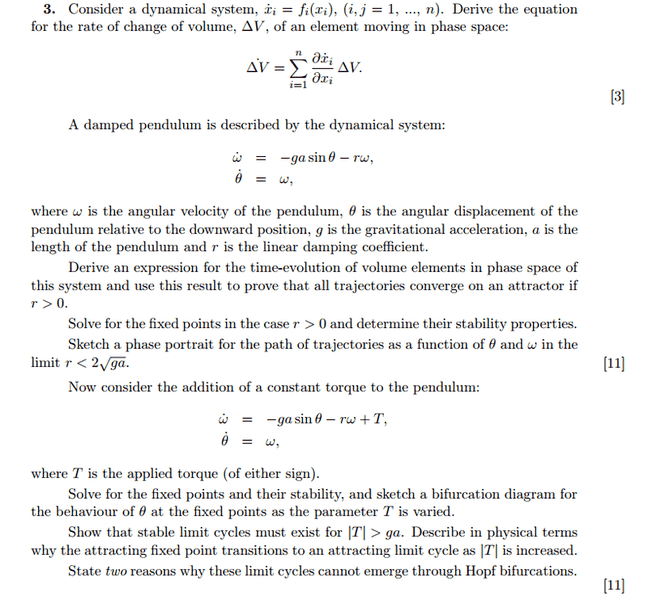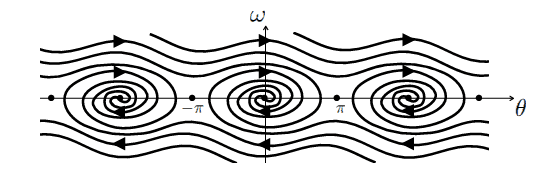# Pendulum - Stability and fixed points

## Homework Statement

(a): Show the lagrangian derivative in phase space
(b)i: Show how the phase space evolves over time and how they converge
(b)ii: Find the fixed points and stability and sketch phase diagram
(c)i: Find fixed points and stability
(c)ii: Show stable limit cycles exist for T>ga and why they cannot emerge by hopf bifurcations[/B]## The Attempt at a Solution

Part(a)
[/B]
Shown using conservation of rate of flow of mass.

Part(b)i

$$\Delta \dot V = \Delta V \left( \frac{\partial \dot \theta}{\partial \theta} + \frac{\partial \dot \omega}{\partial \omega} \right)$$
$$\Delta \dot V = -r \Delta V$$

$$\Delta V = \Delta V_0 e^{-rt}$$

Thus for ##r>0## system converges to an attractor.

Part (b)ii
For ## \dot \omega = \dot \theta = 0##, fixed points are given by ##\theta_0 = n\pi##.

To find their stability, we find ##|J-\lambda I| = 0##
$$\lambda^2 + \lambda r + ga cos\theta = 0$$
Thus for even ##n##, fixed points are stable attractors and for odd ##n##, fixed points are unstable saddle points.Part (c)i

Fixed points are given by:

$$cos \theta = \sqrt{1- \left( \frac{T}{ga} \right)^2}$$

For their stability, again we find the eigenvalues:

$$\lambda^2 + \lambda r + ga cos\theta = 0$$
$$\lambda = \frac{-r \pm \sqrt{ r^2 - 4ga cos\theta } }{2}$$

Thus for ## r^2 > 4ga \sqrt{1- \left( \frac{T}{ga} \right)^2}##, they are fixed stable attractors. For ## r^2 < \sqrt{1- \left( \frac{T}{ga} \right)^2} ##, they are unstable saddle points.

Part(c)ii

I'm not too sure about this part. I know that for ##T>ga##, ##cos \theta## becomes imaginary. How do I relate that to how the fixed point changes to a limit cycle?